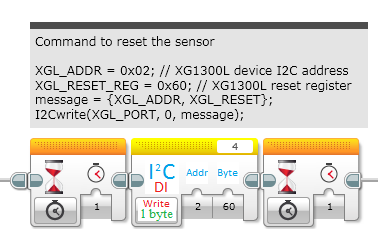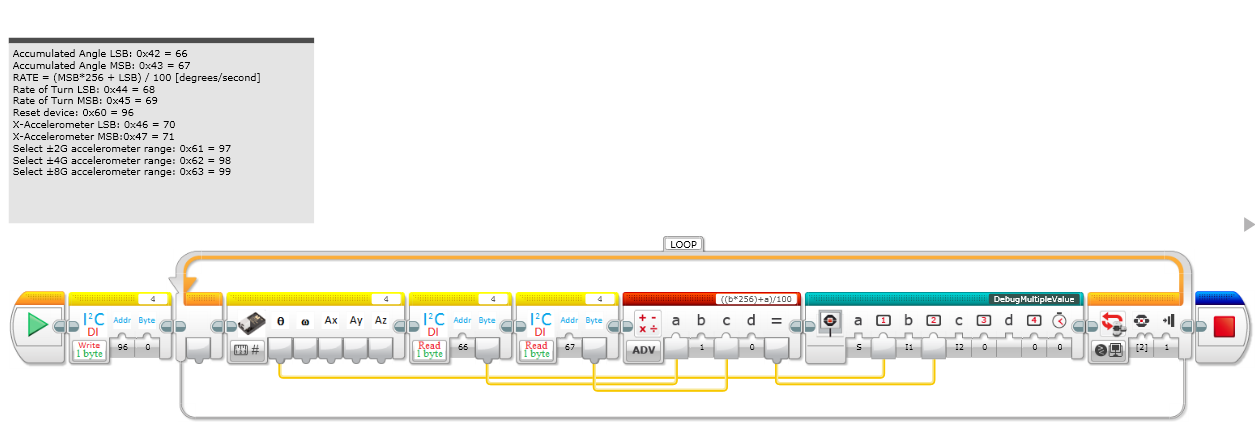# Doubt with the I2C Block [CLOSED]

Hi, in the past, I used the I2C block to stablish a I2C Communication with an Arduino Unit but now, I am trying to use the block to use a way to write and receive values from a Sensor.

When I use the block “Write 1Byte”, the first parameter is the address of the sensor, in this case, normally the sensor are waiting in the address 0x02, so the value is 2, but for the address to write, if the value is 0x60, is it necessary to convert to 7bits or something similar?

Example:Does exist a documentation about the usage of the block?

Related Issues:

Cheers

Juan Antonio

Typically I2C is all 8-bit bytes (there are rare exceptions). The first byte is 7 address bits an one R/W bit. Since the address bits are 1-7 (out of 0-7), the address is sometimes represented left shifted one, and sometimes as the 7 least significant bits (sometimes 1-7, and sometimes 0-6). All other bytes are standard 8 bits (0-7).

After the first address + R/W byte, each following byte can be whatever the system designer decided. How the following bytes are used will be application specific, and in the case of an Arduino, you should be able to configure it however you need (since you can program both the master and slave).

0x02 (hexadecimal) does equal 2 (decimal), but 0x60 does not equal 60. 0x60 (hexadecimal) equals 96 (decimal). Prepending a number with “0x” means it’s hexadecimal i.e. base 16 (as opposed to the default being decimal, which is base 10).

Hi Matt,

many thanks for the reply. today I tested again using your notes and I started reading the values of a Sensor using I2C Registers. This link was a help:
http://www.neurophys.wisc.edu/comp/docs/ascii/Many thanks.

Cheers

Juan Antonio